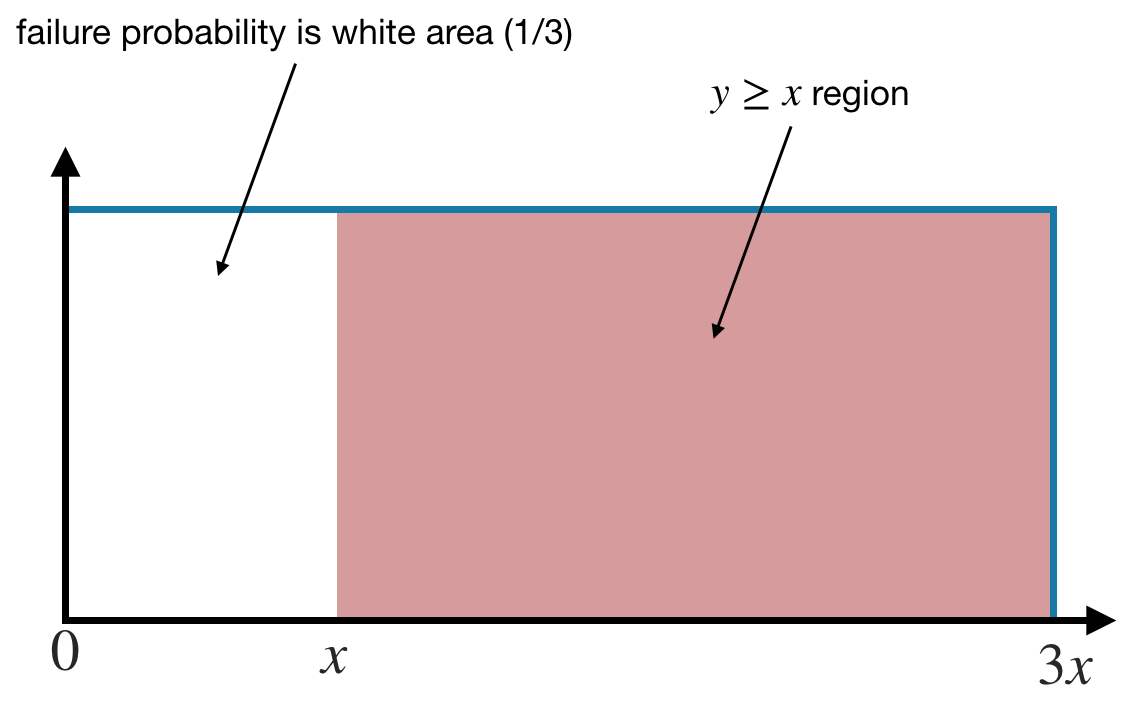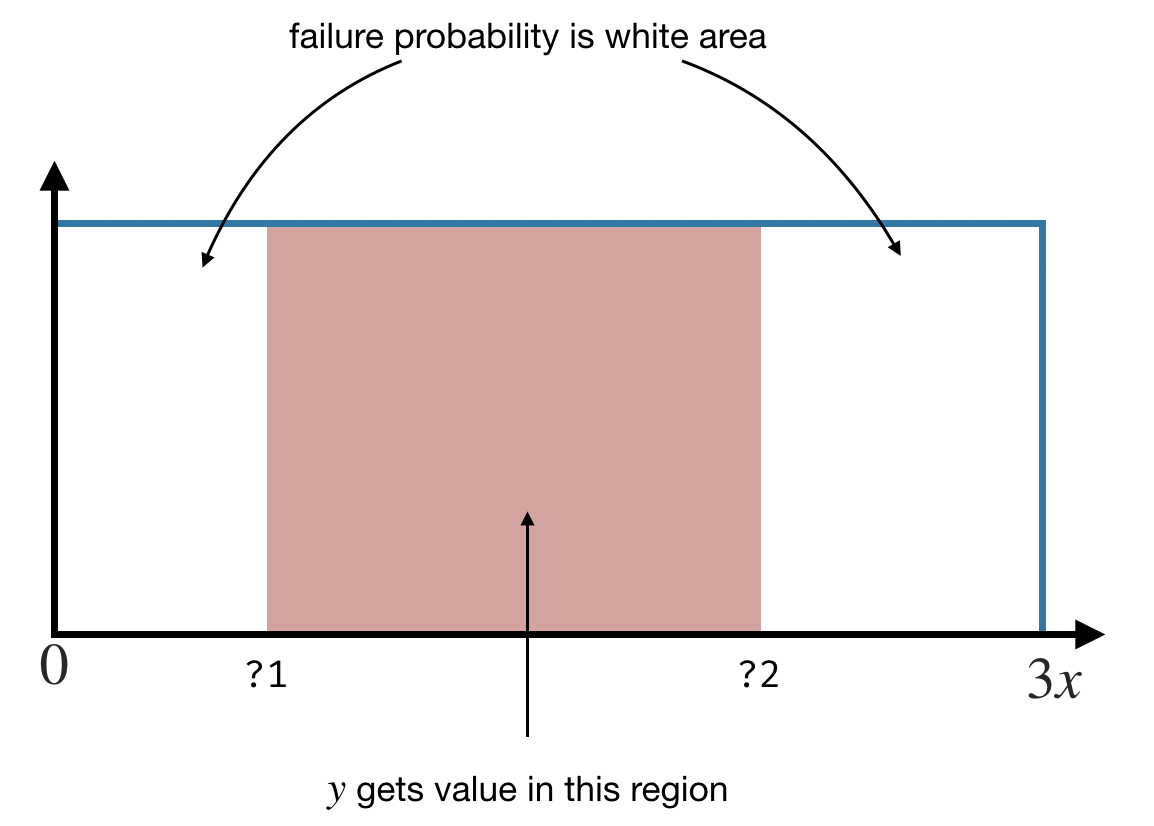Teaching Your SMT Solver Probability Theory
Aws Albarghouthi

The unexpected rise of SAT and SMT solvers has revolutionized software verification, both the automated and deductive flavors. Simply, you encode program semantics as logical circuits and ask the SMT solver questions about them. How elegant. But what happens when your program is randomized? Good luck! The first-order world of SMT solvers does not have the ingredients to sustain your stochastic existence. Go find another home.

In this post, I will show you how to teach your SMT solver probability theory. The ideas here are a simplified view of a recent POPL paper by my student Calvin Smith.

## Classical verification

To ground things, I will start with a classical (non-probabilistic) verification problem and encode it as a formula in first-order logic. Take this program:

def f(x):
z = x + 1
y = z * 2
return y


If we’re working with infinite-precision integers, the following Hoare triple is valid — a positive input results in a positive output.

$\vdash \{x > 0\} ~f(x)~ \{y > 0\}$

To formally prove that this Hoare triple holds, we encode the precondition, postcondition, and program semantics as the following formula:

$(\underbrace{x > 0}_{\text{pre}} \land \underbrace{z = x + 1 \land y = z * 2}_{\text{encoding of } f \ (\text{strongest post})}) \Longrightarrow \underbrace{y > 0}_{\text{post}}$

If the SMT solver tells you that the formula is valid, then the Hoare triple is valid. If it’s not valid, the SMT solver will give you a counterexample.

## Randomized algorithm example

Let’s now look at a very simple randomized program, where uniform(a,b) returns a sample from the uniform distribution between the values a and b.

def f(x):
y = uniform(0,3*x)
return y


Say we want to prove the following (probabilistic) Hoare triple:1

$\vdash_{\color{red}{1/3}} \{x > 0\} ~f(x)~ \{y \geq x\}$

Let’s unpack this: If $x$ is positive, then $f$ returns a value of $y \geq x$, but there is at most a $\color{red}{1/3}$ probability of failing to satisfy the postcondition.

This Hoare triple is intuitively valid: values of $y$ are uniformly distributed between $0$ and $3x$, so getting a value of $y \geq x$ has a failure probability of $1/3$.Cool. But we want to automatically establish this Hoare triple with an SMT solver. How? We’ll get rid of probability. Adios!

## Turning sampling into non-determinism

The idea is that the SMT solver needs to only know a few axioms about the probability distributions in order to construct the proof. In our example, the proof relies on the obvious fact that $y \geq x$ with a probability of $2/3$.

If we know this fact, we can transform the program into a non-deterministic version that tracks probability of failure:

def f_nondet(x):
y = pick a value in [x,...,3*x]
w = 1/3
return y,w


We now have a non-probabilistic program: we force y to receive an arbitrary (non-deterministic) value between x and 3*x; but we know that this may not be true with a probability of 1/3, so we store this fact in a new ghost variable w. The transformation relies on the following insight:

1. make whatever assumptions you want about the value of y
2. but remember the probability with which your assumptions might fail

So now we can prove the above Hoare triple $$\vdash_{\color{red}{1/3}} \{x > 0\} ~f(x)~ \{y \geq x\}$$ using the transformed, non-deterministic program instead:

$(\underbrace{x > 0}_{\text{pre}} \land \underbrace{x \leq y \leq 3x \land w = 1/3}_{\text{encoding of } f_\textit{nondet}}) \Longrightarrow (\underbrace{y \geq x}_{\text{post}} \land \underbrace{w \leq \color{red}{1/3}}_{\text{failure prob.}})$

## Picking the right axioms

In our example, we gave the SMT solver exactly the axiom it needs to know about the uniform distribution. But, in general, we want to automatically discover the right axiom to get the proof to go through. Calvin’s insight was that we can see this as a program synthesis problem!

The idea is to use an axiom family and synthesize the appropriate axiom from this family. Check out this parameterized version of f_nondet above:

def f_synth(x):
y = pick a value in [?1,...,?2]
w = 1 - (?2 - ?1) / 3*x
return y,w


?1 and ?2 are two unknown expressions that we want to synthesize; they define the assumption we are making. Depending on what we choose, we will incur a different probability of failure w.So now you can use your favorite program synthesis engine to synthesize values for the unknowns such that the postcondition $$y \geq x$$ is true and and the failure probability $w \leq 1/3$. Say we pick 2*x and 3*x for ?1 and ?2. We get the following program:

def f_synth_inst1(x):
y = pick a value in [2*x,...,3*x]
w = 2/3
return y,w


This satisfies our postcondition — that $y \geq x$ — but with a failure probability of $2/3$, higher than our goal of $1/3$.

Now check out this other instantiation where we set ?1 to 0 and ?2 to 3*x:

def f_synth_inst2(x):
y = pick a value in [0,...,3*x]
w = 0
return y,w


This has a 0 probability of failure: y always is between 0 and 3*x with a probability of 1. But it does not satisfy the postcondition, since y may very well be less than x.

The synthesizer should return the program f_nondet above, which sets ?1 to x and ?2 to 3*x.

## Synthesis problem

To solve the synthesis problem above with an SMT solver, we encode the problem in the form of $$\exists.\forall .\varphi$$:

$\exists ?_1, ?_2 . \forall x,y,z,w .$ $(\underbrace{x > 0}_{\text{pre}} \land \underbrace{?_1 \leq y \leq ?_2 \land w = 1 - (?_2-?_1)/3x }_{\text{encoding of } f_\textit{synth}}) \Longrightarrow (\underbrace{y \geq x}_{\text{post}} \land \underbrace{w \leq \color{red}{1/3}}_{\text{failure prob.}})$

The idea is we want to find ($\exists$) solutions to the unknowns $?_1$ and $?_2$ such that for any execution ($\forall$) where $x>0$ the postcondition holds and the failure probability is no more than $1/3$.

A solution to this problem is one that sets $?_1$ to $x$ and $?_2$ to $3x$, resulting in the program f_nondet above, whose correctness implies the Hoare triple $$\vdash_{\color{red}{1/3}} \{x > 0\} ~f(x)~ \{y \geq x\}$$.

## Conclusion

That’s it. We’ve thrown probability away. Now you can reason about randomized algorithms with first-order logic. But that’s not to say that solving the resulting formulas is easy!

Our paper gives a full-blow, soundness-police-compliant view of this idea – and a lot of implementation details because some of these formulas involve non-linear arithmetic and quantifier alternation. We manage to automatically prove accuracy properties of some sophisticated algorithms from the differential privacy literature. It’s really fascinating how far we can take SMT solvers.

Thanks to Calvin Smith for comments on an earlier draft. I stole the figures from his slides.

1. Notation from union bound logic, a probabilistic Hoare logic due to Barthe et al.